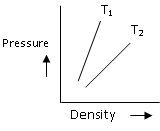#### Figure shows graphs of pressure versus density for an ideal gas at two temperatures T1 and T2 . Which is correct ?Option 1) T1 > T2 Option 2) T1 = T2 Option 3) T1 < T2 Option 4) None of theseAs learnt in

Ideal Gas Law in terms of density -- wherein

where

d - density of gas

P - Pressure

R - Gas Constant

T - Temperature

M - Molar MassTherefore,Option 1)

T1 > T2

correct option

Option 2)

T1 = T2

Incorrect option

Option 3)

T1 < T2

Incorrect option

Option 4)

None of these

Incorrect option